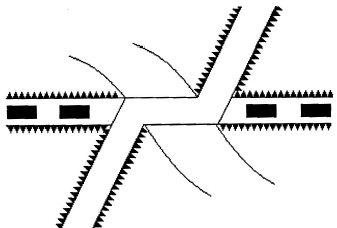Courses

# Test: Surveying- 2

## 25 Questions MCQ Test Civil Engineering SSC JE (Technical) | Test: Surveying- 2

Description
This mock test of Test: Surveying- 2 for Civil Engineering (CE) helps you for every Civil Engineering (CE) entrance exam. This contains 25 Multiple Choice Questions for Civil Engineering (CE) Test: Surveying- 2 (mcq) to study with solutions a complete question bank. The solved questions answers in this Test: Surveying- 2 quiz give you a good mix of easy questions and tough questions. Civil Engineering (CE) students definitely take this Test: Surveying- 2 exercise for a better result in the exam. You can find other Test: Surveying- 2 extra questions, long questions & short questions for Civil Engineering (CE) on EduRev as well by searching above.
QUESTION: 1

Solution:
QUESTION: 2

Solution:
QUESTION: 3

### The line of sight is kept as high above ground surface as possible to minimise the error in the observed angles due to

Solution:
QUESTION: 4

If i is the stadia distance, f is the focal length and d is the distance between the objective and vertical axis of the techeometer, the multiplying constant, is

Solution:
QUESTION: 5

The desired sensitivity of a bubble tube with 2 mm divisions is 30". The radius of the bubble tube should be

Solution:
QUESTION: 6

Subtense bar is an instrument used for

Solution:
QUESTION: 7

Volume of the earth work may be calculated by

Solution:
QUESTION: 8

Tacheometric formula for horizontal distances using horizontal sights can also suitable be

Solution:
QUESTION: 9

Pick up the correct statement from the following:

Solution:
QUESTION: 10

The apparent error on reversal is

Solution:
QUESTION: 11

Over-turning of vehicles on a curve can be avoided by using

Solution:
QUESTION: 12

Which of the following introduces an error of about 1 in 1000 if 20 m chain is used

Solution:
QUESTION: 13

Chain surveying is well adopted for

Solution:
QUESTION: 14

Two contour lines, having the same elevation

Solution:
QUESTION: 15

The conventional sign shown in below figure represents aSolution:
QUESTION: 16

Which of the following statements is incorrect?

Solution:
QUESTION: 17

In levelling operation,

Solution:

In levelling operation if the change point reduced level is known as a control point. Then, taking backsight is not required.

The second sight may be intermediate sight or backsight.

For a  line to be drawn commencement is done with a backsight and closes with foresight in levelling

QUESTION: 18

The length of a traverse leg may be obtained by multiplying the latitude and

Solution:
QUESTION: 19

While working on a plane table, the correct rule is:

Solution:
QUESTION: 20

The vertical angle between longitudinal axis of a freely suspended magnetic needle and a horizontal line at its pivot, is known

Solution:
QUESTION: 21

In the cross-section method of indirect contouring, the spacing of cross-sections depends upon

(i) Contour interval

(ii) Scale of plan

(iii) Characteristics of ground

Solution:
QUESTION: 22

Transition curves are introduced at either end of a circular curve, to obtain

Solution:
QUESTION: 23

Ifa andb be the elevation of two subjects A and B respectively , q be the angle observed by a sextant. The correct horizontal angle is

Solution:
QUESTION: 24

Tilt of the staff in stadia tacheometry increases the intercept if it is

Solution:
QUESTION: 25

Three point problem can be solved by

Solution: